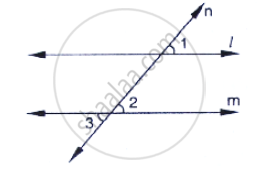# In the Below Fig, ∠1 = 60° And ∠2 = (2/3)^(Rd)Of a Right Angle. Prove that L || M. - Mathematics

In the below fig, ∠1 = 60° and  ∠2 = (2/3)^(rd)of a right angle. Prove that l || m.#### Solution

Given:

∠ = 60°, ∠= (2/3)^(rd) to right angle

To prove: parallel to m

Proof ∠1 = 60°

∠2 = 2/3 × 90° = 60°

Since, ∠1 = ∠2 = 60°

∴ Parallel to m as pair of corresponding angles are equal

Concept: Pairs of Angles
Is there an error in this question or solution?

#### APPEARS IN

RD Sharma Mathematics for Class 9
Chapter 10 Lines and Angles
Exercise 10.4 | Q 9 | Page 47